# Filtering

June 2, 2021

A filter is an operation that discriminates the components of an object over some domain. For example:

• In 3-dimensional space, filtering may include spatial discrimination: rejecting dirt and other large particles while admitting clean water to pass through.
• In the domain of time, Olympic qualifying races use temporal discrimination: rejecting slower runners while admitting faster ones.

### Filtering

In statistics, filtering applies statistical discrimination. For example, a median-5 filter admits the median value of every set of five samples and rejects the other four. These filters are applicable to image processing and financial analysis.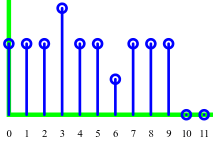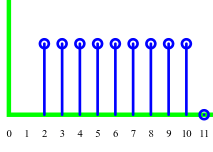Input: 222322122200 Output: 2222222220

In the frequency domain, filtering applies frequency discrimination. Filters reject or accept data components based on how frequently they change.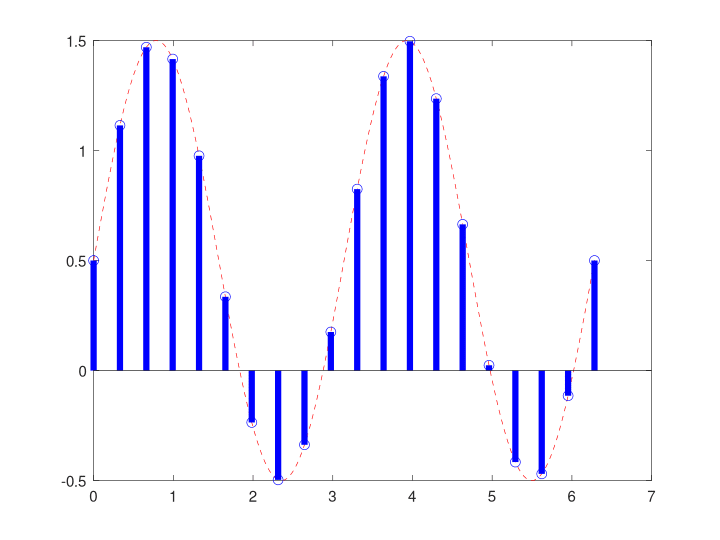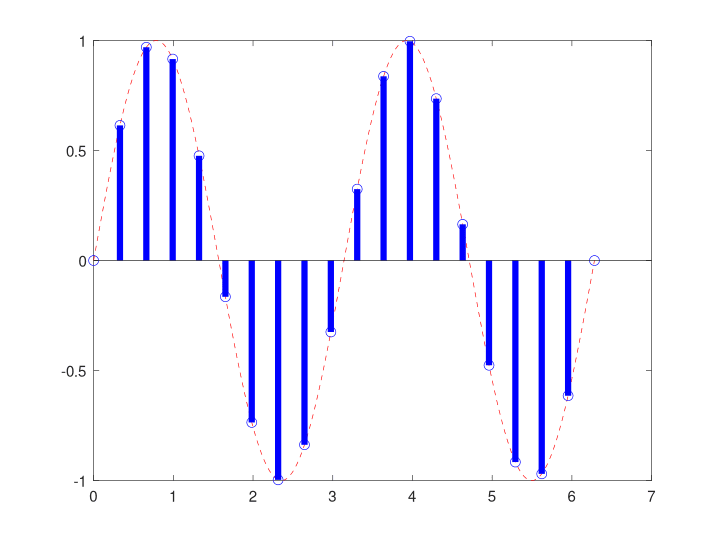Input: Sinusoid + DC Output: Sinusoid only

### Frequency Discriminating Filters

Apply a filter when you want to discriminate data based on frequency.

General examples include:

• Rejecting (eliminate or filter out) frequency components.
• Accepting (admit or pass) frequency components.
• Boosting the energy level (magnitude) of some components.

Specific examples include:

• Filtering out a DC offset (a constant offset)
• Filtering (notching) out 60Hz noise
• Filtering out components outside of a microphone, accelerometer, or other transducers’ specifications
• Filtering out components outside of an audio speaker or other shakers’ specifications
• Equalizing an audio recording by attenuating some frequency bands and boosting others
• Smoothing a noisy data sequence by attenuating rapidly changing (high-frequency) components

### Types of Filter Operations

A filter performs some operation on a data sequence to yield a new sequence. The operation could be anything (or nothing in the case of an identity operation). However, there are some filters that engineers use so frequently that they have a title.

#### Low-Pass Filter

A low-pass filter attenuates high-frequency components above a specified corner frequency (fc) and allows low-frequency components below fc to pass through.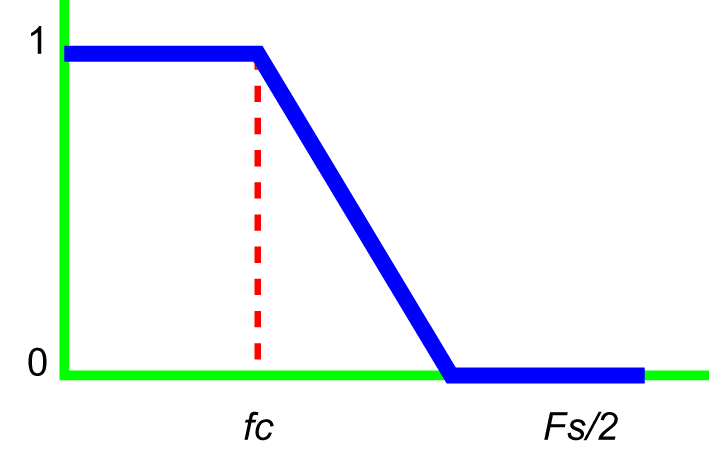For example, a low-pass filter can clean up (or smooth) data contaminated with noise so that patterns are more readily apparent.

#### High-Pass Filter

A high-pass filter attenuates low-frequency components below a specified corner frequency and allows the high-frequency components above it to pass through.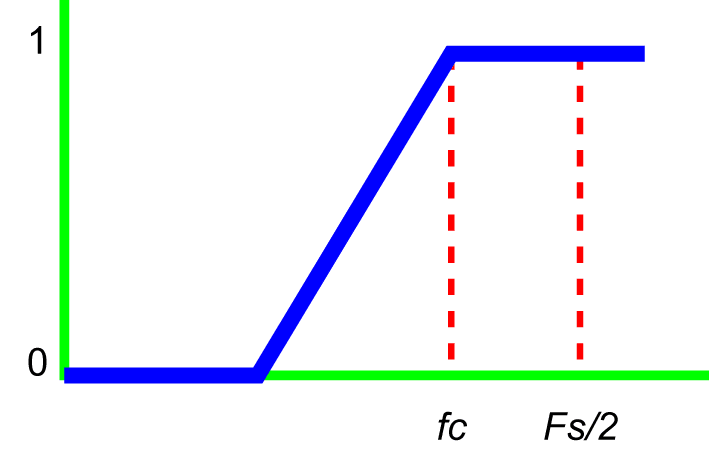For example, if data have a large DC offset, a high-pass filter with a small corner frequency can remove the offset so that patterns of interest are more readily apparent.

#### Bandpass Filter

A bandpass filter attenuates low and high frequencies and allows the middle frequencies to pass through.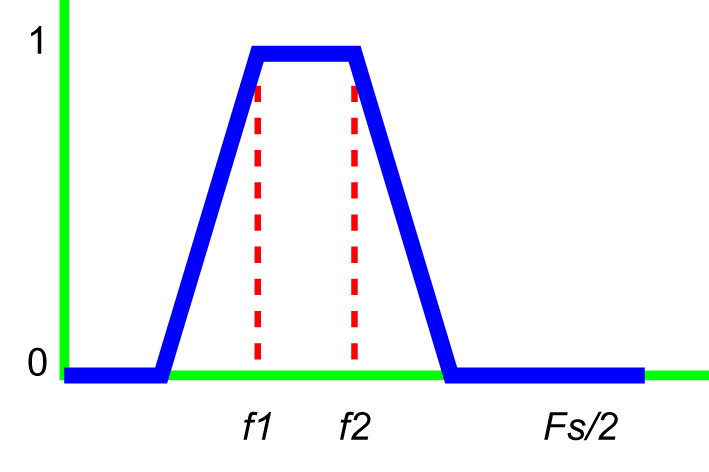#### Notch Filter

A notch filter is the reverse of a bandpass filter. A notch filter rejects a band of frequencies and accepts everything outside the rejected band.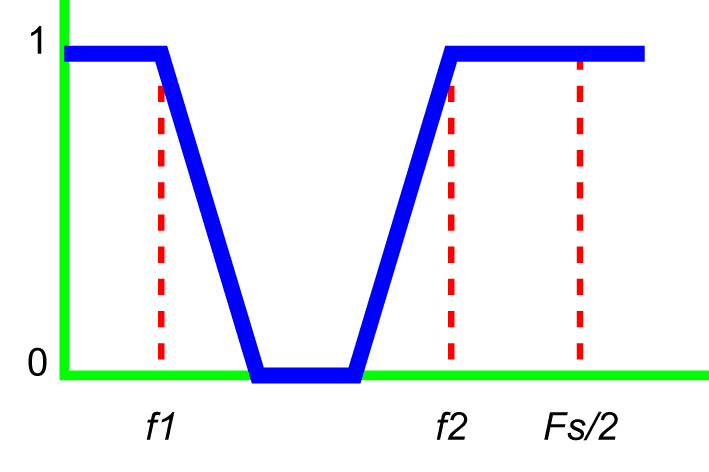### Filter Characteristics

#### Corner Frequency

Typically, the corner frequency of a filter response H(ω) is the frequency ωc, where the spectral power |H(ω)|2 has dropped from |H(ω)|2 = 1 to |H(ω)|2 = ½ or |H(ω)|=1/√2.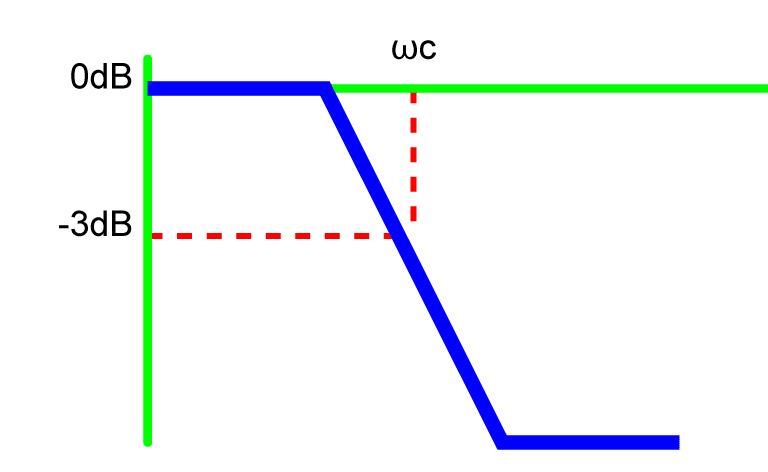On a decibel (dB) scale, the spectral power drops from 10log10(1) = 0dB to:

(1)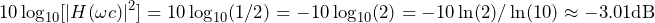#### Roll-Off

There are no perfect filters in the real world—that is, none drop straight down after reaching their corner frequency. Instead, they roll off at some slope. Real-world filters in the frequency domain are not like cliffs but like hills. This hill-like roll-off at the end leaves the upper 5% frequencies of the data invalid.

### Filter Architectures

Mathematics and engineering often synthesize filtering using polynomials.

Mathematics: By the Weierstrass approximation theorem, any continuous function on a closed interval (0 ≤ time ≤ 10) can be approximated to arbitrary precision using polynomials.

Engineering: A polynomial can be implemented with addition and multiplication only. It is easy to implement both operations using digital logic gates in CPUs, DSPs, FPGAs, ASICs, etc.

For example, y = 5x2 + 3x + 2 means:

• Multiply 5 and x and x to get 5x2
• Multiply 3 and x to get 3x
• Add 5x2 and 3x and 2 to get y

Filters are often designed as polynomials over z, where z-n represents a delay by n samples. Therefore, the z-n factors can be implemented into hardware with a simple memory buffer.

#### Example

If a filter in the z-domain has the transfer function:

(2)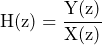(3)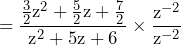(4)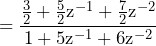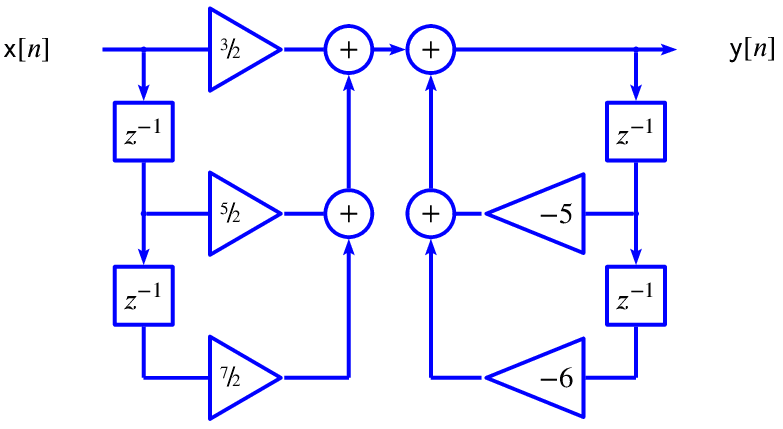Then, in the z-domain:

(5)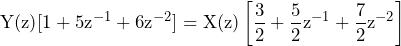In the time domain:

(6)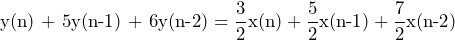Therefore, at time n, the filter output y(n) equals:

(7)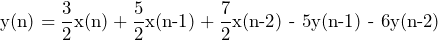### FIR-IIR Comparison

Under the constraint of a polynomial-based filter structure, there are two common filter architectures: FIR filters and IIR filters.#### FIR Filters

The finite impulse response (FIR) filter is a single polynomial. It is the weighted sum of the current input and a finite number of past inputs.

• Extremely stable
• Can be designed to have a linear phase
• Has a finite impulse response: the output will go to exactly zero within a fixed time of the input going to zero

• Tends to introduce long latency into the signal processing chain
• Commonly requires many mathematical operations per input data sample (high computation)

#### IIR Filters

The infinite impulse response (IIR) filter is a polynomial divided by a polynomial (a rational expression). It is the weighted sum of the current input, a finite number of past inputs, and a finite number of past outputs.

• Stable if properly designed but possibly unstable if poorly designed
• Typically requires few mathematical operations per input data sample
• Can be designed to have a linear phase

• Generally has a non-linear phase
• Has an infinite impulse response: generally and theoretically, the output will never reach exactly zero once excited with an input due to the feedback from the output

#### Example

The example below compares an IIR Butterworth 6th order to FIR filters with 17, 33, and 257 coefficients. All have a target corner frequency of 100Hz. Observe the following:

• The 17 coefficient FIR under-performs the 13–15 coefficient Butterworth.
• The 33 coefficient FIR is more-or-less comparable to the Butterworth.
• The 257 coefficient FIR outperforms the Butterworth in terms of roll-off.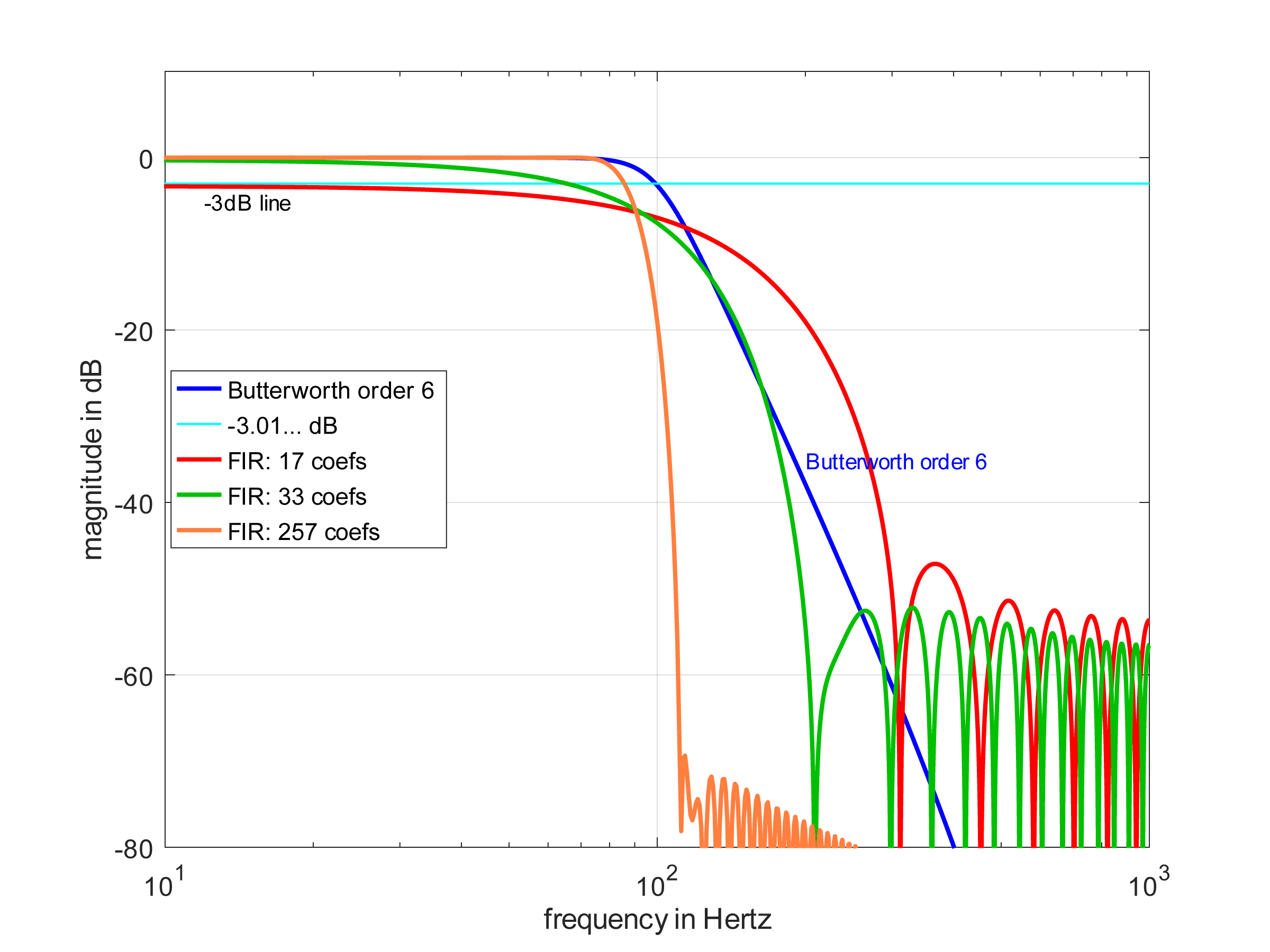An FIR filter with many coefficients introduces a significant delay into the signal processing path as illustrated in the 257-coefficient FIR below.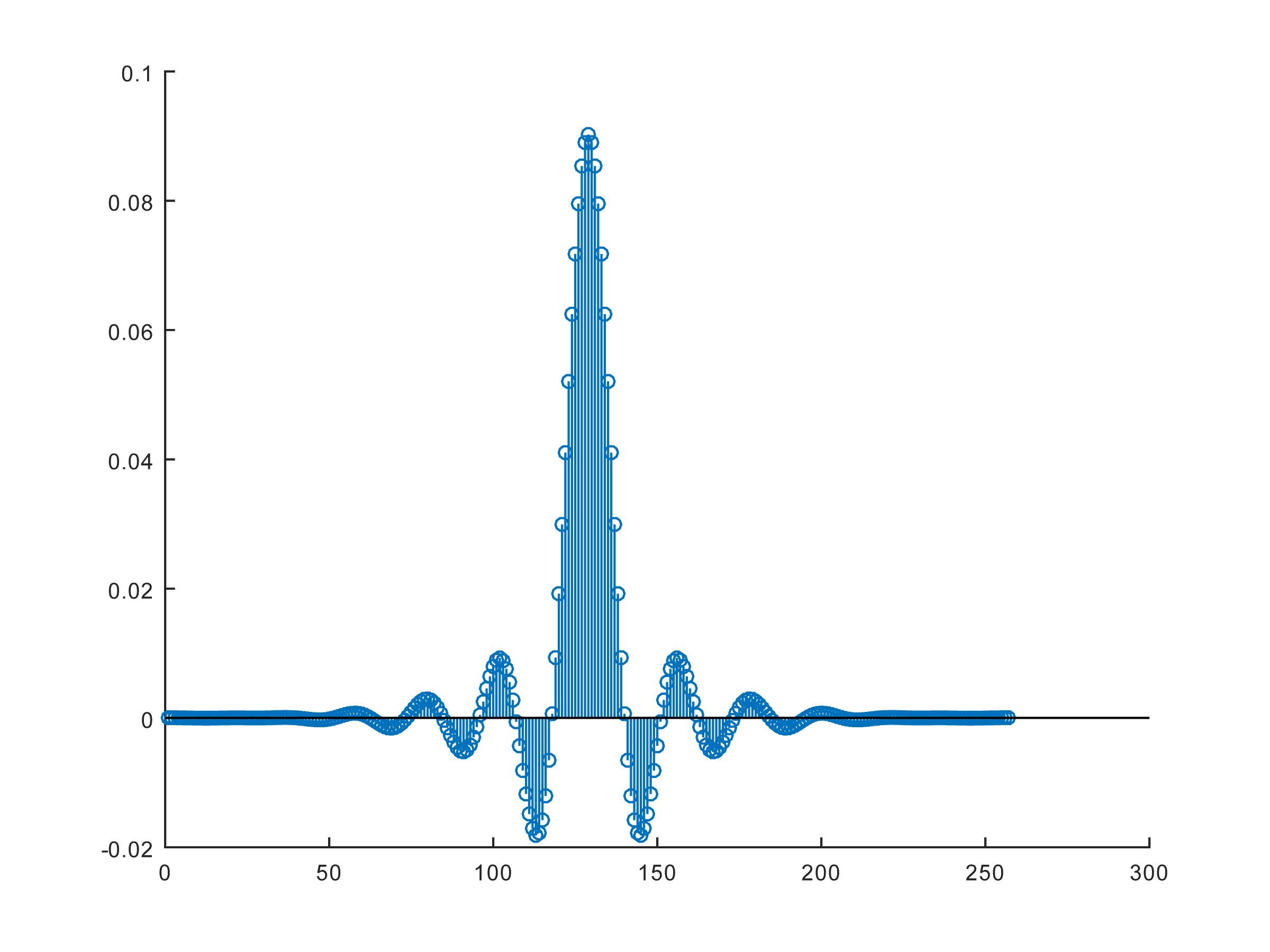FIR filters can easily be designed to have a linear phase. For example, all FIR filters with symmetric coefficients have a linear phase (as illustrated above).

This is not the case with IIR filters, as illustrated with the IIR phase responses illustrated below. However, the Butterworth filter phase responses are approximately linear.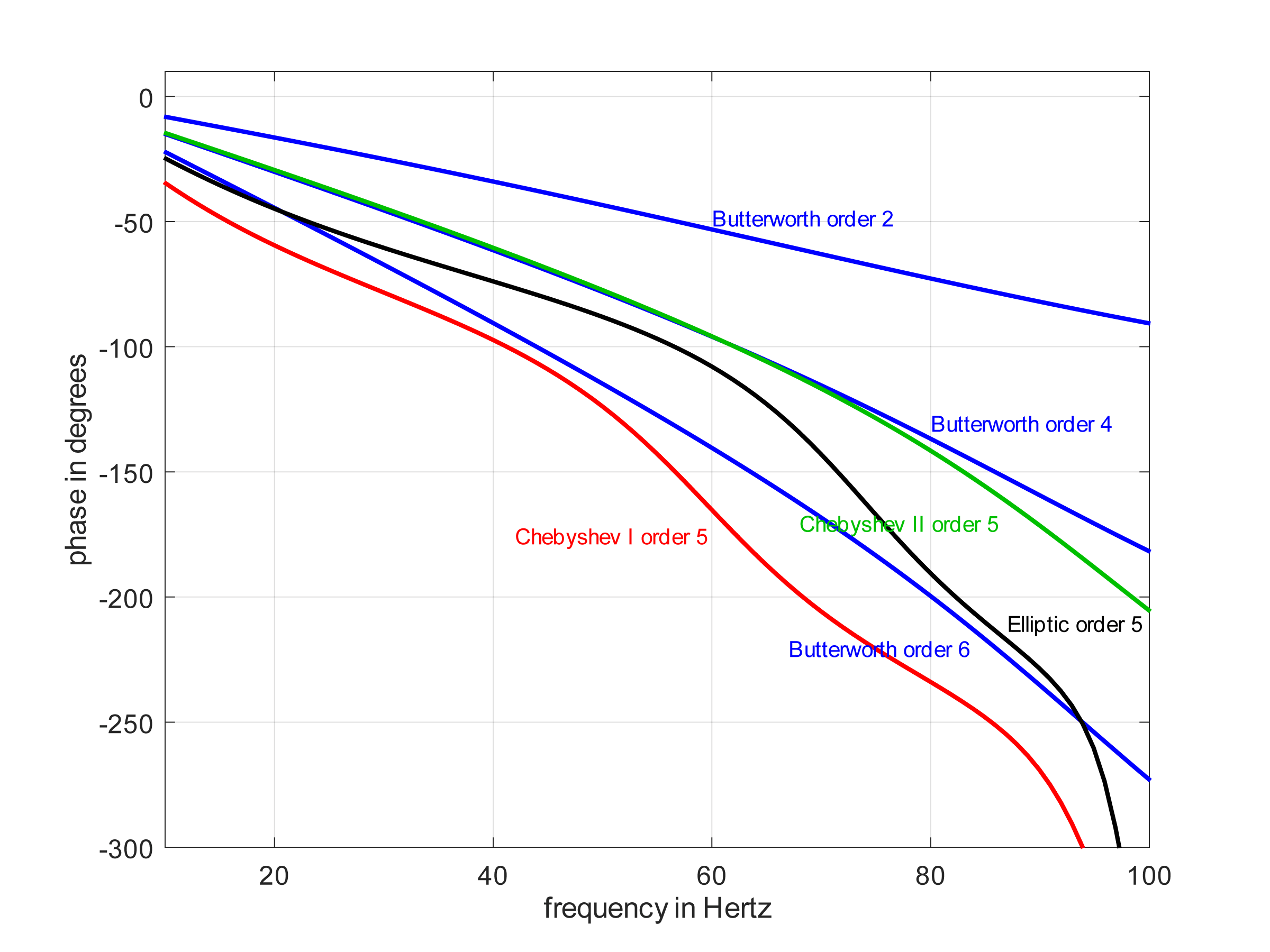### How Filters Work

#### Filtering in Time with Convolution

Often, a computer uses simple arithmetic to perform frequency-domain filtering. Specifically, each output y(n) is often a linear combination of inputs x(n). This type of filtering is called convolution.

For example, suppose we want to filter out direct current (DC). A simple filter with input x(n) and output y(n) will set y(n) = x(n) – x(n-1). Then, any DC signal at the input (x(n) = constant) will be filtered out because y(n) = constant – constant = 0.

Note: this filter is a digital differentiation.

#### Filtering in Frequency with Multiplication

Engineers may also filter a data sequence by first projecting it onto a basis. Often, this basis is a sequence of sines and cosines, and the tool for the projection is the fast Fourier transform (FFT).

According to the convolution theorem, filtering data in the time domain can be performed in the frequency domain by simple point-by-point multiplication. Before the multiplication in frequency can be carried out, we must first transform the data from time to frequency with the FFT.X(f) is the Fourier transform of x(n).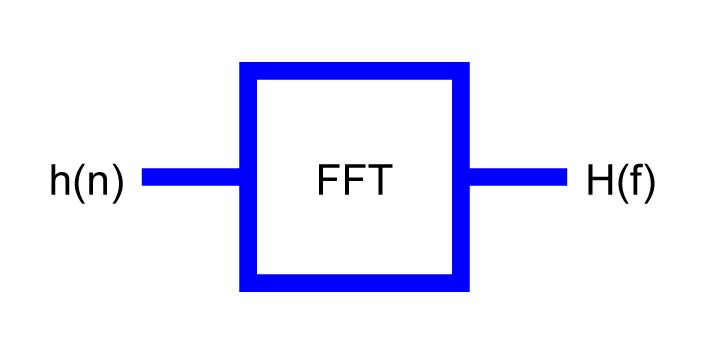H(f) is the Fourier transform of h(n).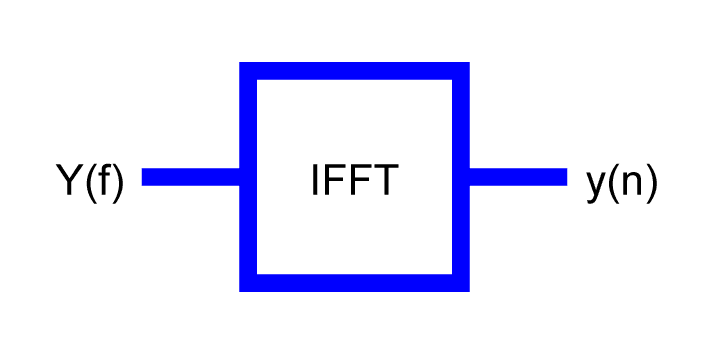y(n) is the inverse Fourier transform of Y(f).